# Subtraction Within 20

Times up!
Times up! You may finish answering the current question and then proceed to your score!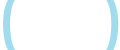Sorry, you have used all of your available hints for this lesson.
Practice Limit Reached

You've reached your daily practice limit of 12 questions.

Award InformationAwarded toQuestion HintChoose Family Member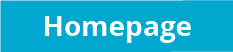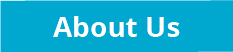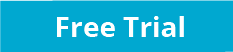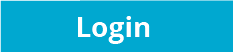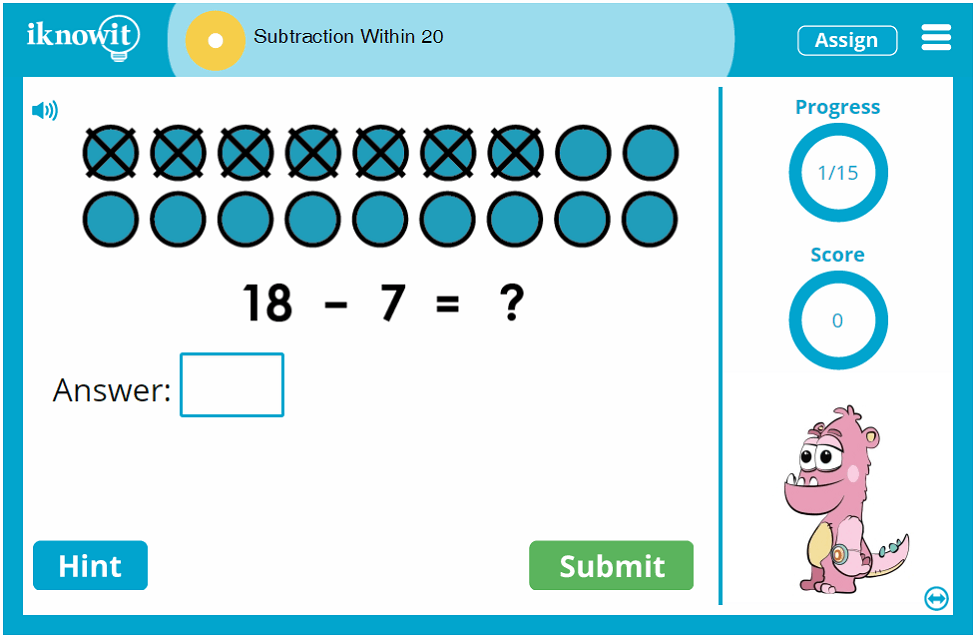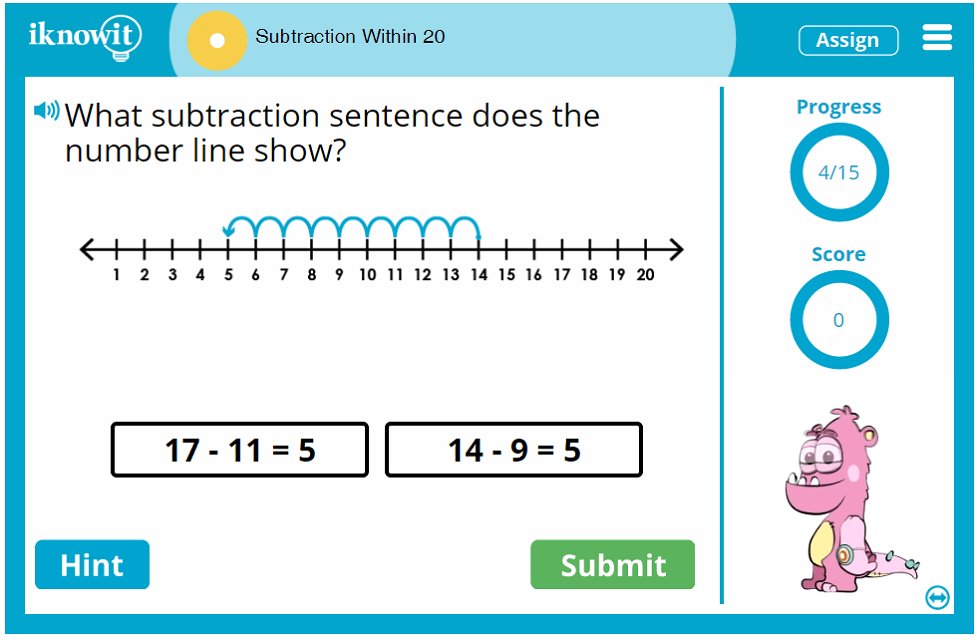## Math Practice Lesson - Subtraction within 20

Are you ready to practice basic subtraction within twenty? This lesson will help your students become subtraction experts! All subtraction problems in this basic math lesson have minuends and subtrahends less than 20. To find the difference, students will be presented with a variety of question types.

Some problems feature a set of counters. Some of the counters have X's through them to represent the part that is taken away, or the subtrahend. The counters are accompanied by a subtraction number sentence. Other problems are word problems. Here's an example of a subtraction word problem your students might be given: 'Emily made 12 snowballs. 3 melted. How many does she have now?' Still other problems might be presented as word problems accompanied by a number line, such as: 'What subtraction sentence does the number line show?' Students will either type their answer in the blank space or choose from multiple choices.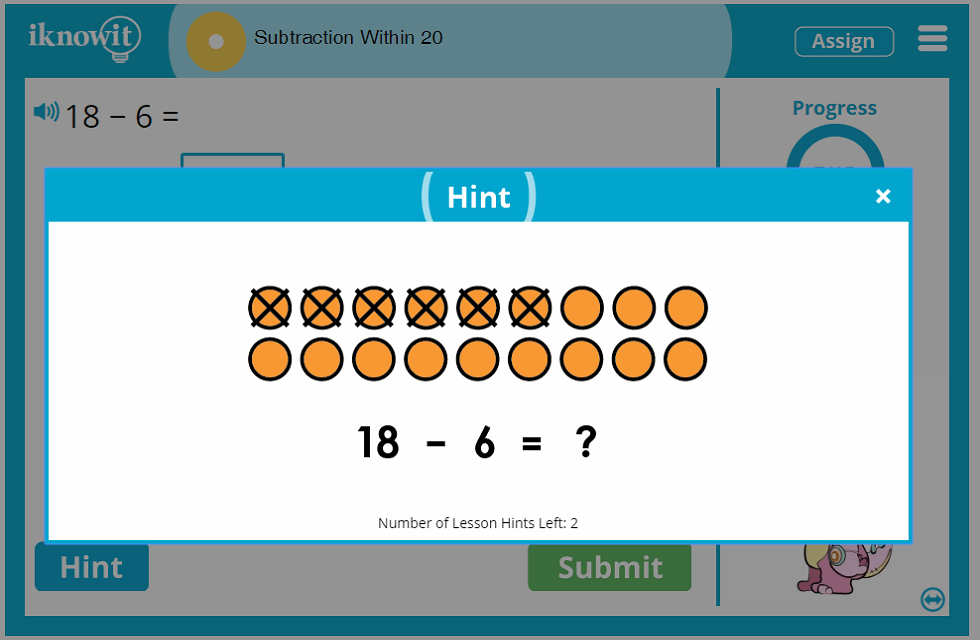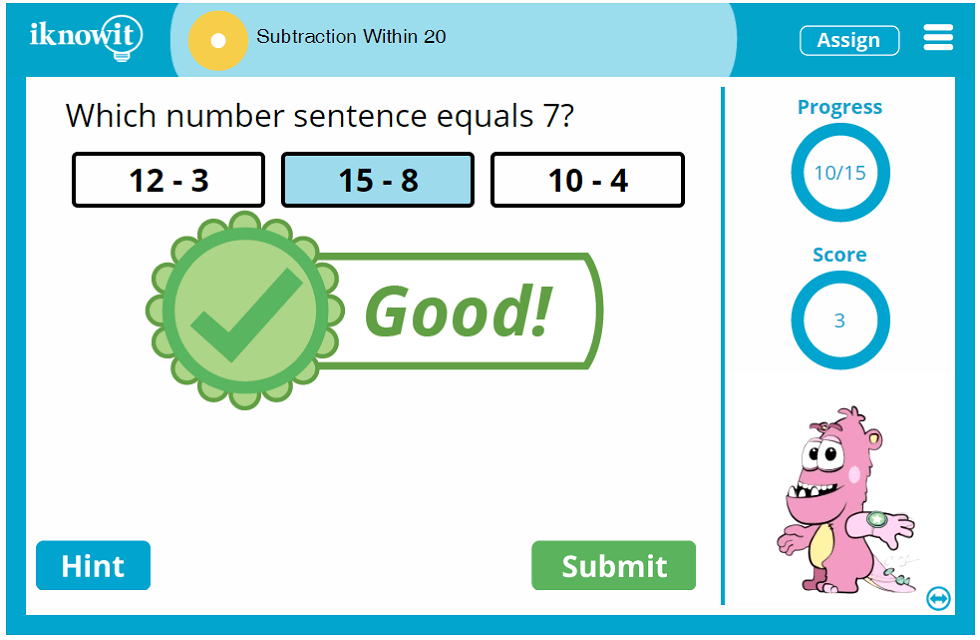## More about I Know It

Math practice should be both educational and fun! That's the inspiration behind iKnowIt.com. Students practice basic math skills with our high quality elementary math content and feel like they are playing a fun (yet challenging) math game. Our kid-friendly, easy-to-navigate website is designed to appeal to both teachers and students! With bright colors, whimsical animated characters, and large icons, iKnowIt makes it very easy to jump right into online math practice!

The math lessons have several features that help students make the most of their math practice time. A progress-tracker on the right side of the screen helps students keep track of how many questions they've answered in their math lesson so far. A score-tracker lets students know how many questions they've answered correctly so far. The hint button in the lower-left corner gives students an optional clue, either written or pictorial, that will help them solve the math problem. When students answer incorrectly, an explanation page appears to show them, step-by-step, how to solve the problem correctly so they can learn from their mistakes.

Another helpful feature you'll find in this subtracting within 20 lesson (and all math lessons on iKnowIt) is the read-aloud feature. Students can click the speaker button on their screen and the question will be read to them in a clear voice. What a great option for ESL/ELL students and children who are auditory learners!

Try out this subtraction within twenty lesson today! We hope you like it! Make sure you also browse our complete collection of other math topics too!

## Become a Member Today!

Did you know you can sign up for a free sixty-day trial of iKnowIt.com? You can! It's the perfect opportunity to use this subtraction lesson with your students and see what 'I Know It' is all about. Students may practice for free, but they will be limited to a total of 25 math questions per day across all 'I Know It' lessons in the free trial mode. For complete and unlimited access, you will need to pay for a subscription to the website.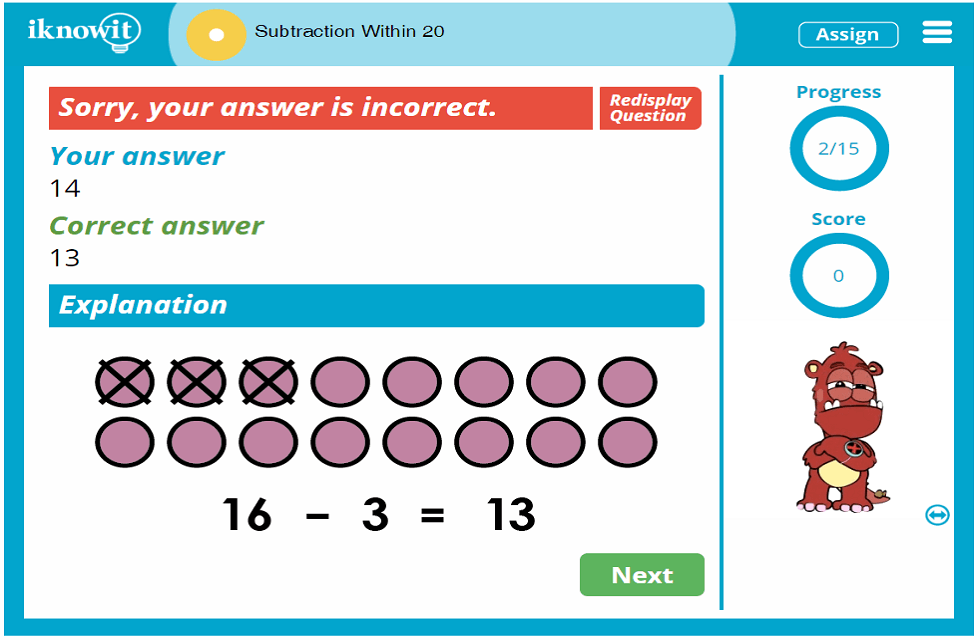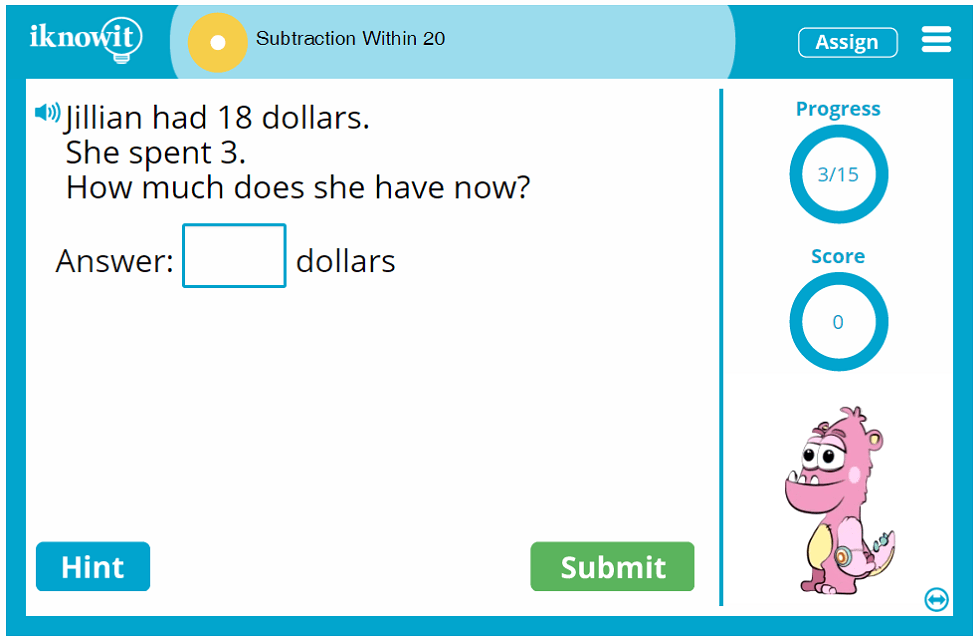## Level

This interactive math lesson is considered a Level A lesson. It may be ideal for first grade students.

## Common Core Standard

1.OA.1
Operations and Algebraic Thinking

Students will represent and solve problems that involve addition and subtraction. They will be able to use subtraction within twenty to solve word problems involving situations of adding to, taking away from, putting together, taking apart, and comparing with the aid of objects, drawings, and equations with a symbol for the unknown number to represent the problem.

## You might also be interested in...

Subtraction Fact Drills within Ten (Level A)
In this first grade-level math lesson, students will practice subtracting within ten. Questions are presented in a drill-style format.

Subtraction Fact Drills within Twenty (Level A)
Students will practice subtracting within twenty in this first grade-level math lesson. Questions are presented in a drill-style format.